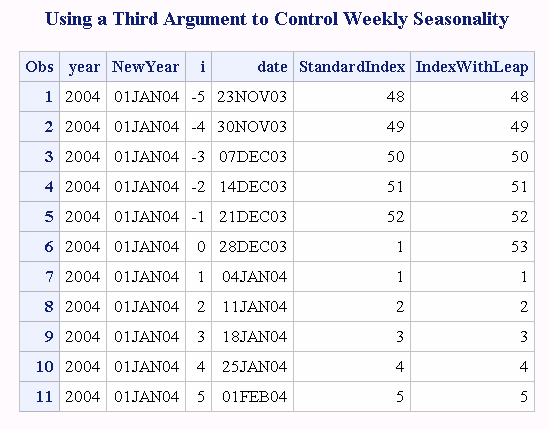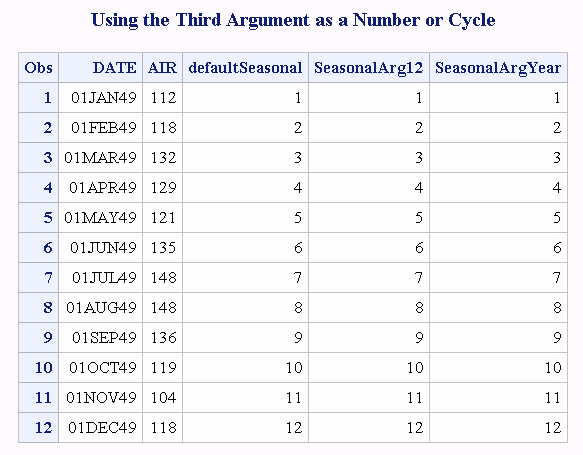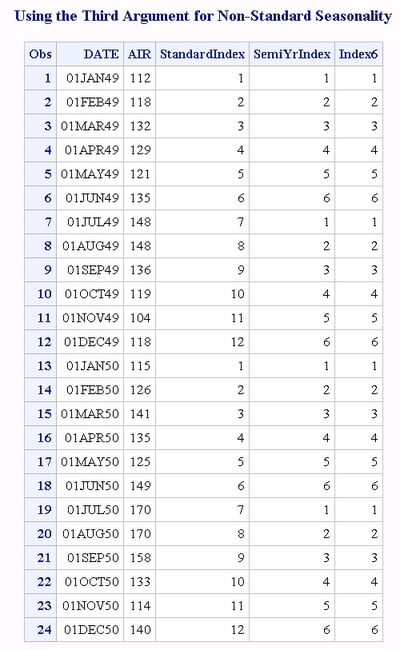# INTINDEX Function

Returns the seasonal index when a date, time, or datetime interval and value are specified.

 Category: Date and Time

## Syntax

INTINDEX(interval<<multiple. > >, date-value, )

### Required Arguments

#### interval

specifies a character constant, a variable, or an expression that contains an interval name such as WEEK, MONTH, or QTR. Interval can appear in uppercase or lowercase. The possible values of interval are listed in Intervals Used with Date and Time Functions in SAS Language Reference: Concepts.

Tip
If interval is a character constant, then enclose the value in quotation marks.
Tip
Valid values for interval depend on whether date-value is a date is a date, time, or datetime value. For more information, see .
Multipliers and shift indexes can be used with the basic interval names to construct more complex interval specifications. The general form of an interval name is listed below:

#### interval<multiple.shift-index>

The three parts of the interval name are as follows:

#### interval

specifies the name of the basic interval type. For example, YEAR specifies yearly intervals.

#### multiple

specifies an optional multiplier that sets the interval equal to a multiple of the period of the basic interval type. For example, the interval YEAR2 consists of two-year, or biennial, periods.

 See Incrementing Dates and Times by Using Multipliers and by Shifting Intervals for more information.

#### shift-index

specifies an optional shift index that shifts the interval to start at a specified subperiod starting point. For example, YEAR.3 specifies yearly periods shifted to start on the first of March of each calendar year and to end in February of the following year.

 Restrictions The shift index cannot be greater than the number of subperiods in the whole interval. For example, you could use YEAR2.24, but YEAR2.25 would be an error because there is no 25th month in a two-year interval. If the default shift period is the same as the interval type, then only multiperiod intervals can be shifted with the optional shift index. For example, because MONTH type intervals shift by MONTH subperiods by default, monthly intervals cannot be shifted with the shift index. However, bimonthly intervals can be shifted with the shift index, because there are two MONTH intervals in each MONTH2 interval. For example, the interval name MONTH2.2 specifies bimonthly periods starting on the first day of even-numbered months. See Incrementing Dates and Times by Using Multipliers and by Shifting Intervals for more information.

#### date-value

specifies a date, time, or datetime value that represents a time period of the given interval.

### Optional Argument

#### seasonality

specifies a number or a cycle.

This argument enables you to have more flexibility in working with dates and time cycles. You can specify whether you want a 52-week or a 53-week seasonality in a year.
 Example In the following example, the function `INTINDEX('MONTH', date, 3);` produces the same result as `INTINDEX('MONTH', date, 'QTR');`Seasonality in the first example is a number (the number of months), and in the second example seasonality is a cycle (QTR).

## Details

### INTINDEX Function Intervals

The INTINDEX function returns the seasonal index when you supply an interval and an appropriate date, time, or datetime value. The seasonal index is a number that represents the position of the date, time, or datetime value in the seasonal cycle of the specified interval. For example, `intindex('month', '01DEC2000'd);` returns a value of 12 because there are 12 months in a yearly cycle and December is the 12th month of the year. In the following examples, INTINDEX returns the same value because both statements have values that occur in the first quarter of the year 2000: `intindex('qtr', '01JAN2000'd);` and ```intindex('qtr', '31MAR2000'd);```. The statement `intindex('day', '01DEC2000'd);` returns a value of 6 because daily data is weekly periodic and December 1, 2000, is a Friday, the sixth day of the week.

### How Interval and Date-Time-Value Are Related

To correctly identify the seasonal index, the interval should agree with the date, time, or datetime value. For example, ```intindex('month', '01DEC2000'd);``` returns a value of 12 because there are 12 months in a yearly interval and December is the 12th month of the year. The MONTH interval requires a SAS date value. In the following example, ```intindex('day', '01DEC2000'd);``` returns a value of 6 because there are seven days in a weekly interval and December 1, 2000, is a Friday, the sixth day of the week. The DAY interval requires a SAS date value.
The example `intindex('qtr', '01JAN2000:00:00:00'dt);` results in an error because the QTR interval expects the date to be a SAS date value rather than a datetime value. The example `intindex('dtmonth', '01DEC2000:00:00:00'dt);` returns a value of 12. The DTMONTH interval requires a datetime value.

### Retail Calendar Intervals

The INTINDEX function can also be used with calendar intervals from the retail industry. These intervals are ISO 8601 compliant. For more information, see .

### Seasonality

Seasonality is a time series concept that measures cyclical variations at different intervals during the year. In specifying seasonality, the time of year is the most common source of the variations. For example, sales of home heating oil are regularly greater in winter than during other times of the year. Often, certain days of the week cause regular fluctuations in daily time series, such as increased spending on leisure activities during weekends. The INTINDEX function uses the concept of seasonality and returns the seasonal index when a date, time, or datetime interval and value are specified. For more information about seasonality and using the forecasting methods in PROC FORECAST, see the SAS/ETS User's Guide.

## Comparisons

The INTINDEX function returns the seasonal index whereas the INTCINDEX function returns the cycle index.
In the example `index = intindex('day', '04APR2005'd);`, the INTINDEX function returns the day of the week. In the example ```cycle_index = intcindex('day', '04APR2005'd);```, the INTCINDEX function returns the week of the year.
In the example `index = intindex('minute','01Sep78:00:00:00'dt);`, the INTINDEX function returns the minute of the hour. In the example ```cycle_index = intcindex('minute','01Sep78:00:00:00'dt);```, the INTCINDEX function returns the hour of the day.
In the example `intseas('interval');`, INTSEAS returns the maximum number that could be returned by `intindex('interval', date);`.

## Examples

### Example 1: Examples of Using INTINDEX with Two Arguments

The following SAS statements produce these results.
SAS Statement
Result
```interval1 = intindex('qtr', '14AUG2005'd);
put interval1;```
`3`
```interval2 = intindex('dtqtr','23DEC2005:15:09:19'dt);
put interval2;```
`4`
```interval3 = intindex('hour', '09:05:15't);
put interval3;```
`10`
```interval4 = intindex('month', '26FEB2005'd);
put interval4;```
`2`
```interval5 = intindex('dtmonth', '28MAY2005:05:15:00'dt);
put interval5;```
`5`
```interval6 = intindex('week', '09SEP2005'd);
put interval6;```
`36`
```interval7 = intindex('tenday', '16APR2005'd);
put interval7;```
`11`

### Example 2: Example of Seasonality

SAS uses a default seasonal cycle. For example, the assumption is that monthly data is yearly seasonal. However, monthly data could also have a seasonal cycle of semiyearly. This example shows that to use a third argument, seasonality, enables you to specify the seasonality rather than using the default. It also shows how to handle leap years:
```data weekly;
*do year = 2000 to 2010;
year = 2004;
NewYear = HOLIDAY('NEWYEAR',year);
do i = -5 to 5;
date = INTNX('week',NewYear,i);
output;
end;
*end;
format date date.;
format NewYear date.;
run;

/* The standard leap week is the first week of year. */
/* An alternative method uses a third argument:leap week is week 53. */
title "Using a Third Argument to Control Weekly Seasonality";
data LeapWeekExample;
set weekly;
StandardIndex = INTINDEX('week',date);
IndexWithLeap = INTINDEX('week',date,53);
run;
proc print;
run;

/* Using a number and an interval can be equivalent for the third argument. */
title "Using the Third Argument as a Number or Cycle";
data Equiv3rdArg;
set sashelp.air(obs=12);
defaultSeasonal = INTINDEX('MONTH',date);
SeasonalArg12 = INTINDEX('MONTH',date,12);
SeasonalArgYear = INTINDEX('MONTH',date,'YEAR');
format date date.;
run;
proc print;
run;

/* Use the third argument for non-standard seasonality. */
title "Using the Third Argument for Non-Standard Seasonality";
data NonStandardSeasonal;
set sashelp.air(obs=24);
/* Standard Index - MONTH is Yearly Seasonal */
StandardIndex = INTINDEX('MONTH',date);
SemiYrIndex = INTINDEX('MONTH',date,'SEMIYR');
Index6 = INTINDEX('MONTH',date,6);
format date date.;
run;

proc print;
run;
```
Output from the Seasonality Example